### Home > CC2MN > Chapter 2 > Lesson 2.2.4 > Problem2-81

2-81.

Copy and complete each of the Diamond Problems below. The pattern used in the Diamond Problems is shown at right.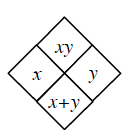1.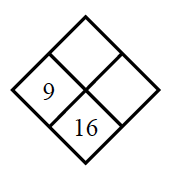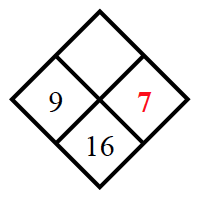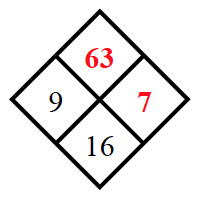Find the number that will complete the expression $9+\underline{\qquad}=16$ . Added to the diamond, 7 in right space.

With the number in red you complete the diamond by finding the product of the two numbers. Added to the diamond, 63 in top space.

$(9)(7)=63$

The numbers $7$ and $63$ complete the diamond.

1.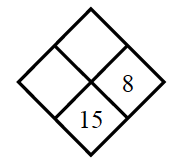This problem is very similar to part (a). Try solving the problem on your own. What added to $8$ gives $15$?

The missing numbers are $7$ and $56$.

1.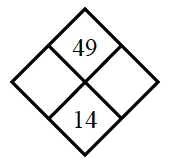Use parts (a) and (b) as guides for solving this problem.
You may also want to try guessing and checking for possible numbers to complete the boxes, since you cannot begin with subtraction or division.

1.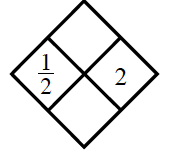To complete the diamond, find the sum and product of the two given numbers.